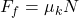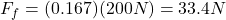An object is known to have a coefficient of kinetic friction (µk) of 0.167 and a coefficient of static friction (µk) of 0.42. If the normal

Question

An object is known to have a coefficient of kinetic friction (µk) of 0.167 and a coefficient of static friction (µk) of 0.42. If the normal force is 200 N, how much frictional force will it encounter while it is moving?

in progress 0
5 months 2021-08-26T19:47:05+00:00 1 Answers 30 views 0

Ff = 33.4N

Explanation:

To find the frictional force while the object is moving, you take into account that the friction force depends of the coefficient of kinetic friction.

The frictional force is given by:(1)

Ff: frictional force = ?

µk: coefficient of kinetic friction = 0.167

N: normal force of the object = 200N

You replace the values of the parameters in the equation (1):The frictional force, while the objects is moving, is 33.4N